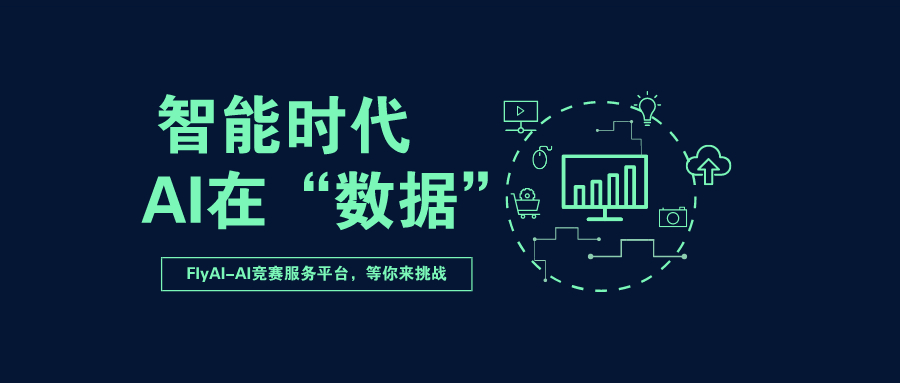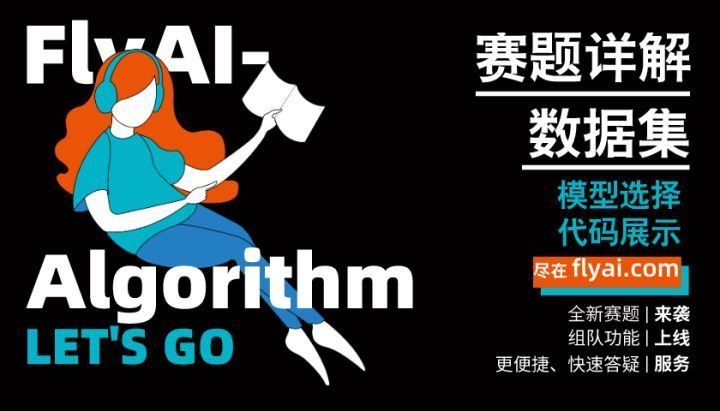# Tensorflow基础课程6：深度强化学习（DRL）,基础教程CartPole 游戏

``````　　import gym

env = gym.make('CartPole-v1') # 实例化一个游戏环境，参数为游戏名称

state = env.reset() # 初始化环境，获得初始状态

while True:

env.render() # 对当前帧进行渲染，绘图到屏幕

action = model.predict(state) # 假设我们有一个训练好的模型，能够通过当前状态预测出这时应该进行的动作

next_state, reward, done, info = env.step(action) # 让环境执行动作，获得执行完动作的下一个状态，动作的奖励，游戏是否已结束以及额外信息

if done: # 如果游戏结束则退出循环

break``````

``````import tensorflow as tf

import numpy as np

import gym

import random

from collections import deque

num_episodes = 500 # 游戏训练的总episode数量

num_exploration_episodes = 100 # 探索过程所占的episode数量

max_len_episode = 1000 # 每个episode的最大回合数

batch_size = 32 # 批次大小

learning_rate = 1e-3 # 学习率

gamma = 1. # 折扣因子

initial_epsilon = 1. # 探索起始时的探索率

final_epsilon = 0.01 # 探索终止时的探索率``````

``````　　class QNetwork(tf.keras.Model):

def __init__(self):

super().__init__()

self.dense1 = tf.keras.layers.Dense(units=24, activation=tf.nn.relu)

self.dense2 = tf.keras.layers.Dense(units=24, activation=tf.nn.relu)

self.dense3 = tf.keras.layers.Dense(units=2)

def call(self, inputs):

x = self.dense1(inputs)

x = self.dense2(x)

x = self.dense3(x)

return x

def predict(self, inputs):

q_values = self(inputs)

return tf.argmax(q_values, axis=-1)

最后，我们在主程序中实现 Q Learning 算法。

if __name__ == '__main__':

env = gym.make('CartPole-v1') # 实例化一个游戏环境，参数为游戏名称

model = QNetwork()

replay_buffer = deque(maxlen=10000) # 使用一个 deque 作为 Q Learning 的经验回放池

epsilon = initial_epsilon

for episode_id in range(num_episodes):

state = env.reset() # 初始化环境，获得初始状态

epsilon = max( # 计算当前探索率

initial_epsilon * (num_exploration_episodes - episode_id) / num_exploration_episodes,

final_epsilon)

for t in range(max_len_episode):

env.render() # 对当前帧进行渲染，绘图到屏幕

if random.random() < epsilon: # epsilon-greedy 探索策略，以 epsilon 的概率选择随机动作

action = env.action_space.sample() # 选择随机动作(探索)

else:

action = model.predict(np.expand_dims(state, axis=0)).numpy() # 选择模型计算出的 Q Value 最大的动作

action = action

# 让环境执行动作，获得执行完动作的下一个状态，动作的奖励，游戏是否已结束以及额外信息

next_state, reward, done, info = env.step(action)

# 如果游戏Game Over，给予大的负奖励

reward = -10. if done else reward

# 将(state, action, reward, next_state)的四元组(外加 done 标签表示是否结束)放入经验回放池

replay_buffer.append((state, action, reward, next_state, 1 if done else 0))

# 更新当前 state大连专业人流医院 http://www.dlrlyy.net/

state = next_state

if done: # 游戏结束则退出本轮循环，进行下一个 episode

print("episode %d, epsilon %f, score %d" % (episode_id, epsilon, t))

break

if len(replay_buffer) >= batch_size:

# 从经验回放池中随机取一个批次的四元组，并分别转换为 NumPy 数组

batch_state, batch_action, batch_reward, batch_next_state, batch_done = zip(

*random.sample(replay_buffer, batch_size))

batch_state, batch_reward, batch_next_state, batch_done = \

[np.array(a, dtype=np.float32) for a in [batch_state, batch_reward, batch_next_state, batch_done]]

batch_action = np.array(batch_action, dtype=np.int32)

q_value = model(batch_next_state)

y = batch_reward + (gamma * tf.reduce_max(q_value, axis=1)) * (1 - batch_done) # 计算 y 值

loss = tf.keras.losses.mean_squared_error( # 最小化 y 和 Q-value 的距离

y_true=y,

y_pred=tf.reduce_sum(model(batch_state) * tf.one_hot(batch_action, depth=2), axis=1)

)Python Free

QQ：417803890